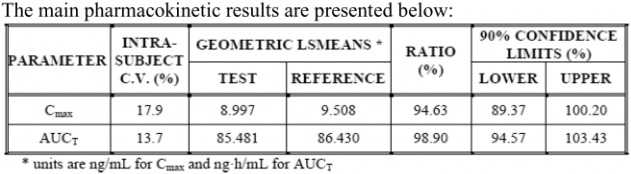## Manipulations with PK data in BE analysis [General Sta­tis­tics]

Dear colleagues.

I would like to know how pharamcokinetic data is manipulated during statistical analysis. Specifically, I would like to know when we should perform ln transformation: we calculate Geom mean and then transform its value to ln value and do ANOVA or we transform each concentration Cmax to Ln-data and then calculate geom mean. Or maybe it is not relevant?
I also would like to know how GMR is usually presented in tables. Whether it is usually GMR of ln-transformed or non-transformed data? I provide the table below as an example and would like to know the origin of its values as I asked above.Ing. Helmut Schütz